# The Kernel Cookbook:

### Update: I've turned this page into a chapter of my thesis.

If you've ever asked yourself: "How do I choose the covariance function for a Gaussian process?" this is the page for you. Here you'll find concrete advice on how to choose a covariance function for your problem, or better yet, make your own.

If you're looking for software to implement Gaussian process models, I recommend GPML for Matlab, or GPy for Python. These software packages deliberately do not provide a default kernel. You might ask: "These guys surely know more about GPs than me, why don't they include a sensible default?"

The answer is that the choice of kernel (a.k.a. covariance function) determines almost all the generalization properties of a GP model. You are the expert on your modeling problem - so you're the person best qualified to choose the kernel! If you don't yet know enough about kernels to choose a sensible one, read on.

### Support Vector Machines

If your question is: "How do I choose a kernel for a Support Vector Machine"? Then a lot of the advice below might still be helpful, especially the first section on standard kernels. However, if you want to construct an interesting composite kernel, you'll probably have a hard time learning all the parameters by cross-validation. This is why most SVM kernels have only one or two parameters.

## Standard Kernels

### Squared Exponential KernelA.K.A. the Radial Basis Function kernel, the Gaussian kernel. It has the form:
$$k_{\textrm{SE}}(x, x') = \sigma^2\exp\left(-\frac{(x - x')^2}{2\ell^2}\right)$$
Neil Lawrence says that this kernel should be called the "Exponentiated Quadratic". The SE kernel has become the de-facto default kernel for GPs and SVMs. This is probably because it has some nice properties. It is universal, and you can integrate it against most functions that you need to. Every function in its prior has infinitely many derivatives. It also has only two parameters:
• The lengthscale $$\ell$$ determines the length of the 'wiggles' in your function. In general, you won't be able to extrapolate more than $$\ell$$ units away from your data.
• The output variance $$\sigma^2$$ determines the average distance of your function away from its mean. Every kernel has this parameter out in front; it's just a scale factor.$$k_{\textrm{RQ}}(x, x') = \sigma^2 \left( 1 + \frac{(x - x')^2}{2 \alpha \ell^2} \right)^{-\alpha}$$
This kernel is equivalent to adding together many SE kernels with different lengthscales. So, GP priors with this kernel expect to see functions which vary smoothly across many lengthscales. The parameter $$\alpha$$ determines the relative weighting of large-scale and small-scale variations. When $$\alpha \rightarrow \infty$$, the RQ is identical to the SE.

#### Pitfalls of the SE and RQ kernels

Most people who set up a GP regression or classification model end up using the Squared-Exp or Rational Quadratic kernels. They are a quick-and-dirty solution that will probably work pretty well for interpolating smooth functions when N is a multiple of D, and when there are no 'kinks' in your function. If your function happens to have a discontinuity or is discontinuous in its first few derivatives (for example, the abs() function), then either your lengthscale will end up being extremely short, and your posterior mean will become zero almost everywhere, or your posterior mean will have 'ringing' effects. Even if there are no hard discontinuities, the lengthscale will usually end up being determined by the smallest 'wiggle' in your function - so you might end up failing to extrapolate in smooth regions if there is even a small non-smooth region in your data.

If your data is more than two-dimensional, it may be hard to detect this problem. One indication is if the lengthscale chosen by maximum marginal likelihood never stops becoming smaller as you add more data. This is a classic sign of model misspecification.

### Periodic Kernel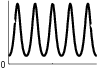$$k_{\textrm{Per}}(x, x') = \sigma^2\exp\left(-\frac{2\sin^2(\pi|x - x'|/p)}{\ell^2}\right)$$
The periodic kernel (derived by David Mackay) allows one to model functions which repeat themselves exactly. Its parameters are easily interpretable:
• The period $$p$$ simply determines the distnace between repititions of the function.
• The lengthscale $$\ell$$ determines the lengthscale function in the same way as in the SE kernel.

### Locally Periodic Kernel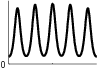A SE kernel times a periodic results in functions which are periodic, but which can slowly vary over time.
$$k_{\textrm{LocalPer}}(x, x') = k_{\textrm{Per}}(x, x')k_{\textrm{SE}}(x, x') = \sigma^2\exp\left(-\frac{2\sin^2(\pi|x - x'|/p)}{\ell^2}\right) \exp\left(-\frac{(x - x')^2}{2\ell^2}\right)$$
Most periodic functions don't repeat themselves exactly. To add some flexibility to our model, we can consider adding or multiplying a local kernel such as the squared-exp with our periodic kernel. This will allow us to model functions that are only locally periodic - the shape of the repeating part of the function can now change over time.

### Linear Kernel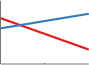$$k_{\textrm{Lin}}(x, x') = \sigma_b^2 + \sigma_v^2(x - c)(x' - c)$$
If you use just a linear kernel in a GP, you're simply doing Bayesian linear regression, and good news! You can do this in time $$\mathcal{O}(N)$$ instead of $$\mathcal{O}(N^3)$$, so you should probably go use software specifically designed for that.

We include the linear kernel here because further on, we'll show you how to combine it with other kernels in order to get some nice properties.

The linear kernel is not like the others in that it's non-stationary. A stationary covariance function is one that only depends on the relative position of its two inputs, and not on their absolute location. That means that the parameters of the linear kernel are about specifying the origin:
• The offset $$c$$ determines the x-coordinate of the point that all the lines in the posterior go though. At this point, the function will have zero variance (unless you add noise)
• The constant variance $$\sigma_b^2$$ determines how far from 0 the height of the function will be at zero. It's a little confusing, becuase it's not specifying that value directly, but rather putting a prior on it. It's equivalent to adding an uncertain offset to our model. See What about the mean function?

## Combining Kernels

The kernels above are useful if your data is all the same type, but what if you have more than one type of feature, but you still want to regress on all of them together? The standard way to build a kernel over different datatypes is to multiply kernels together.

### Multiplying Kernels

Multiplying together kernels is the standard way to combine two kernels, especially if they are defined on different inputs to your function.
Roughly speaking, multiplying two kernels can be thought of as an AND operation. That is, if you multiply together two kernels, then the resulting kernel will have high value only if both of the two base kernels have a high value. Here are a few examples, in addition to the squared-exp times periodic above:

#### Linear times PeriodicA linear kernel times a periodic results in functions which are periodic with increasing amplitude as we move away from the origin.

#### Linear times LinearA linear kernel times another linear kernel results in functions which are quadratic! This trick can be taken to produce Bayesian polynomial regression of any degree.

#### Multidimensional Products

Multiplying two kernels which each depend only on a single input dimension results in a prior over functions that vary across both dimesions. That is, the function value $$f(x,y)$$ is only expected to be similar to some other function value $$f(x',y')$$ if $$x$$ is close to $$x'$$ AND $$y$$ is close to $$y'$$.

These kernels have the form: $$k_{\textrm{product}}(x, y, x', y') = k_x(x, x') k_y(y, y')$$

Here we show both a multimensional product kernel, and a draw from the corresponding GP prior:Roughly speaking, adding two kernels can be thought of as an OR operation. That is, if you add together two kernels, then the resulting kernel will have high value if either of the two base kernels have a high value.

#### Linear plus PeriodicA linear kernel plus a periodic results in functions which are periodic with increasing mean as we move away from the origin.

Adding kernels which each depend only on a single input dimension results in a prior over functions which are a sum of one-dimensional functions, one for each dimension. That is, the function $$f(x,y)$$ is simply a sum of two functions $$f_x(x) + f_y(y)$$.

These kernels have the form:
$$k_{\textrm{additive}}(x, y, x', y') = k_x(x, x') + k_y(y, y')$$

Here we show both an additive kernel, and a draw from the corresponding GP prior:One nice thing about constructing an additive function is that you can decompose your posterior over functions into additive parts. That is, if the kernel is a sum
$$k_{\textrm{sum}}(x, x') = k_1(x, x') + k_2(x, x') + \dots + k_D(x, x')$$, then your posterior can also be decomposed into a sum of Gaussian processes, each with mean
$$\textrm{Mean}(f_d(x^\star)) = k_d( x^\star, X) K_{\textrm{sum}}(X, X)^{-1} f(X)$$
and variance
$$\textrm{Cov}(f_d(x^\star), f_d(x^\star)) = k_d( x^\star, x^\star) - k_d( x^\star, X) K_{\textrm{sum}}(X, X)^{-1} k_d( X, x^\star)$$

## Discrete Data

Kernels can be defined over all types of data structures: Text, images, matrices, and even kernels . Coming up with a kernel on a new type of data used to be an easy way to get a NIPS paper.

### How to use categorical variables in a Gaussian Process regression

There is a simple way to do GP regression over categorical variables. Simply represent your categorical variable as a by a one-of-k encoding. This means that if your number ranges from 1 to 5, represent that as 5 different data dimensions, only one of which is on at a time.

Then, simply put a product of SE kernels on those dimensions. This is the same as putting one SE ARD kernel on all of them. The lengthscale hyperparameter will now encode whether, when that coding is active, the rest of the function changes. If you notice that the estimated lengthscales for your categorical variables is short, your model is saying that it's not sharing any information between data of different categories.

## Other Types of Structure

### How to encode symmetry into the kernel

In some cases, we know that the function we're modeling is symmetric. There turns out to be an simple trick to ensure that all the functions you consider are symmetric: simply add the kernel to itself, but with the order of the inputs swapped.

#### Axis-aligned reflective symmetry

For example, to enforce that $$f(x) = f(-x)$$, simply transform your kernel like so: $$k_{\textrm{1d_Symmetry}}(x, x') = k(x, x') + k(-x, x')$$

#### Enforcing independence from the order of arguments

To enforce that $$f(x,y) = f(y,x)$$, use this kernel transformation: $$k_{\textrm{2d_Symmetry}}(x, y, x', y') = k(x, y, x', y') + k(y, x, x', y')$$
Here's an example of a function drawn from such a symmetric GP prior: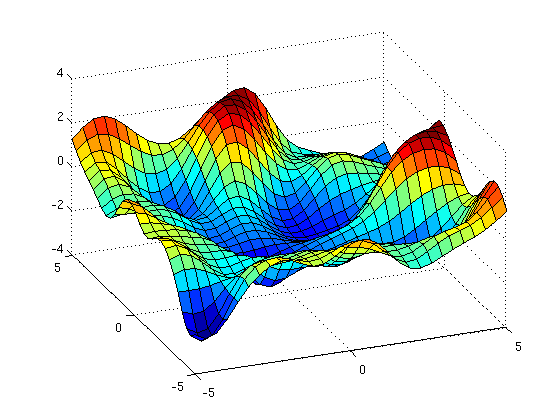[code to generate this figure]

If you want to include symmetry among more arguments, you'll have to include more permutations.

### How to encode low dimensional structure

Unless there is a lot of smoothness, or some regularity in your function, doing high-dimensional regression can be very hard. [Actually, often you are saved by the fact that your data actually live on a low-dimensional manifold, so that you only need to learn the function on a small, smooth subspace!] However, sometimes we might wish to assume that our high-dimensional function is actually low-dimensional in some sense. This is most easily done by regressing on a low-dimensional projection of our inputs. Perhaps you will not be surprised that you can accomplish this by modifying your kernel:

If you want to enforce that your function is low-dimensionsal, use the following transformation: $$k_{\textrm{low-D}}(x, x') = k(Ax, Ax')$$ where $$A$$ is a low-rank matrix.
Here is an example of just such a low-rank kernel, and a draw from the corresponding GP prior: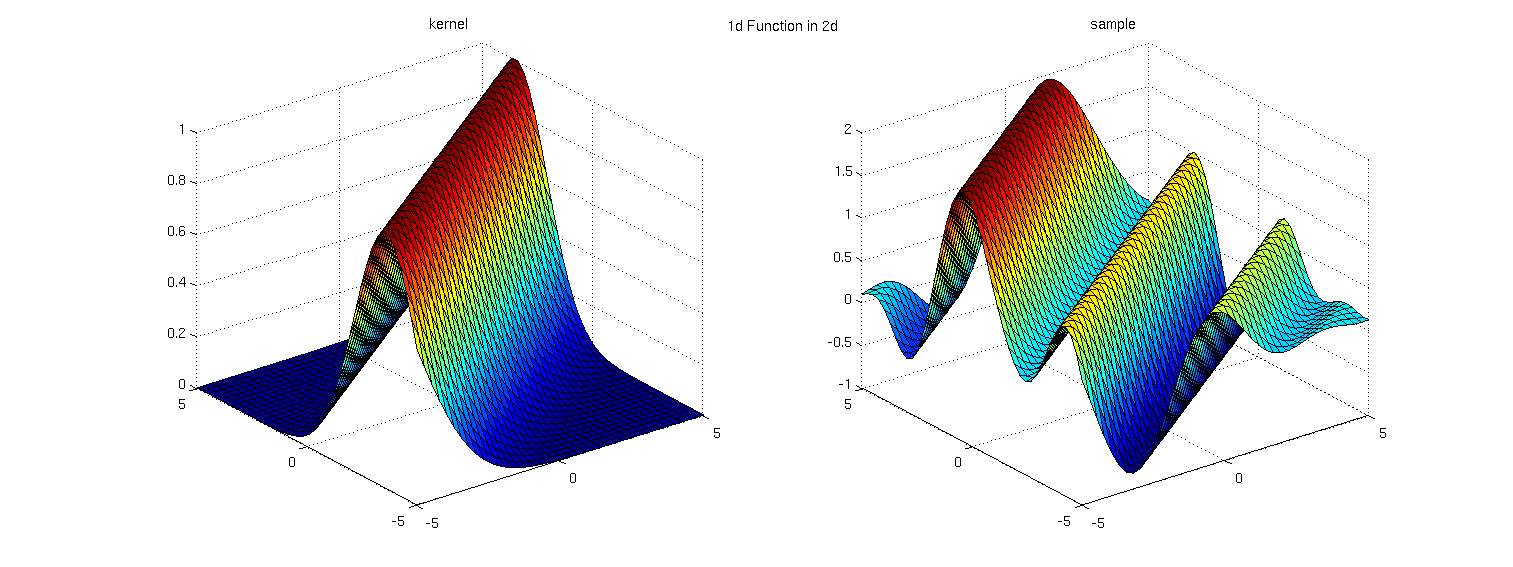Of course, we might want to allow that our function is only mostly low-dimensional. In that case, we can add a small, local, high-dimensional component to our kernel to allow it to vary locally a little bit: $$k_{\textrm{low-D}}(x, x') = k(Ax, Ax') + k(x,x')$$Another variation (from Carl's book) is to include the extra variance in the linear mapping, which has a form similar to factor analysis:
$$k_{\textrm{low-D}}(x, x') = k((A + I)x, (A + I)x')$$:These approaches were written about in a paper about sparse GP regression: Variable noise and dimensionality reduction for sparse Gaussian processes by Edward Snelson and Zoubin Ghahramani. They point out that learning the low-dimensional projection as hyperparameters of your kernel is preferable to simply doing GP regression after doing PCA, since PCA doesn't actually know anything about function being modeled (the values $$f(X)$$) - it only knows about the data distribution $$X$$.

### What about the mean function?

You can integrate out linear and constant mean functions exactly, provided you have zero-mean Gaussian priors on their parameters. However, it is usually a good idea to optimize an empirical constant mean function. This can be interpreted as empirically setting the mean of the prior on your constant mean function.

## Automatically Choosing a Kernel

After reading all this advice, you might decide that you're not sure which kernel is approriate for your problem. In fact, you might decide that choosing the kernel is one of the main difficulties in doing inference - and just as you don't know what the true parameters are, you also don't know what the true kernel is. Probably, you should try out a few different kernels at least, and compare their marginal likelihood on your training data.

However, it might be annoying to write down all the different kernels you want to try, especially if there are more than a few variations you're interested in. If want to let the computer run a search over kernels for you, we've made code available to do just that. We wrote about these automatic searches in a paper.